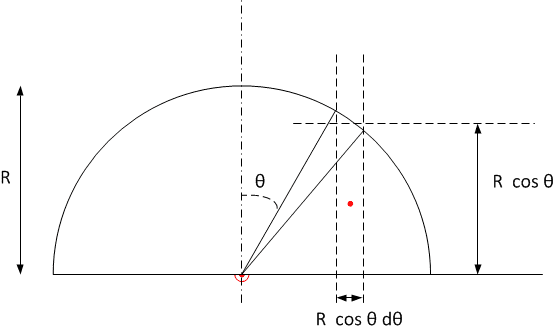# COM of a half circle

Physgeek64

## Homework Statement

Find the centre of mass of a semi-circle

## Homework Equations

##y_{cm}=\frac{1}{M} \int y dm ##

## The Attempt at a Solution

So ## y= R cos \theta ## where theta is measured from the vertical, and the base of the semi-circle is along the horizontal

Now apparently from here you can change coordinated to polar coordinates and replace ##dm## with ## \rho r dr d \theta## to obtain the correct answer of ##\frac {4}{3 \pi}## But I'm confused as to why you can't also replace ##dm## with ##2 \rho R sin \theta R d \theta## to split to 'dm' segment into rows perpendicular to the horizontal base?

Many thanks :)

## Answers and Replies

Homework Helper
With ##R\cos\theta## the height of such a slice, what would you have for the width ? I would guess ##\ R\cos\theta\ d\theta\ ## And the center of mass of such a slice would be at ##{R\over 2} \cos\theta##. It might work. Make a drawing to check what you are doing.

Physgeek64
With ##R\cos\theta## the height of such a slice, what would you have for the width ? I would guess ##\ R\cos\theta\ d\theta\ ## And the center of mass of such a slice would be at ##{R\over 2} \cos\theta##. It might work. Make a drawing to check what you are doing.

Hi- I did draw it out, but unfortunately cannot scan it in. The best way I can describe what Im doing is by saying that I'm calculating it in the same way I would the moment of inertia by dividing the semicircle into rectangles of length ## 2 R sin \theta## (or ## sin \theta## depending on how you define your angles) and width ##Rd \theta## It does not appear to give the correct answer....

Homework Helper
Actuallly it does give the correct answer. Could you show at least your calculation ?But: at first you mentioned vertical rectangles.
And you had already defined ##\theta##; why change it ?

Last edited:
Staff Emeritus
R‧dΘ is the arc length, so it's a sloping distance perpendicular to neither axis.

Mentor
I think it would be better to set this up in cartesian coordinates, and then to convert to polar coordinates in doing the trig substitution as part of the integration. If y is the distance above the base, the area of the differential "rectangle" between y and y + dy is ##2\sqrt{R^2-(R-y)^2}dy##. The moment of the area about the base is ##2y\sqrt{R^2-(R-y)^2}dy##. This can be integrated from y = 0 to y = R to get the total moment of the semi-circle.

Physgeek64
Actuallly it does give the correct answer. Could you show at least your calculation ?

View attachment 101612

But: at first you mentioned vertical rectangles.
And you had already defined ##\theta##; why change it ?

Hi- sorry if I was not being very clear. This is what I had meant :) but I can't see my mistake

#### Attachments

Staff Emeritus
The rectangular strip has its long side of length 2‧R‧cosθ
but its dimension perpendicular to this is .....

Physgeek64
The rectangular strip has its long side of length 2‧R‧cosθ
but its dimension perpendicular to this is .....
Oh okay so it should be ##dy= Rcos \theta d \theta ## in place of ##R d \theta## ? :)

Physgeek64
The rectangular strip has its long side of length 2‧R‧cosθ
but its dimension perpendicular to this is .....

And it comes out with that correction! Thank you so much :)

Staff Emeritus